MORE IN Structural Analysis - 1
GTU Civil Engineering (Semester 3)
Structural Analysis - 1
December 2014
Total marks: --
Total time: --
INSTRUCTIONS
(1) Assume appropriate data and state your reasons
(2) Marks are given to the right of every question
(3) Draw neat diagrams wherever necessary

1 (a) Find out SI and KI of the structures shown in the fig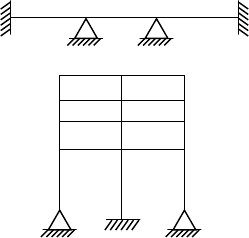4 M
1 (b) Define Influence Line Diagram and give it's uses.
3 M
1 (c) Draw the S.F and B.M diagram for the beam loaded as shown in the fig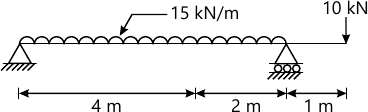7 M

2 (a) A train of loads 35 kN, 30 kN & 25 kN, at a distance 2 m from each other, crosses a simply supported girder of span18m from left to right. Calculate maximum SF& BM at section 6 m from left.
7 M
2 (b) Draw influence line diagram for RA , MA & Mx for cantilever beam having span 6 m and point X is at 2 m from support A.
7 M
2 (c) Draw the S.F and B.M diagram for the frame loaded as shown in the fig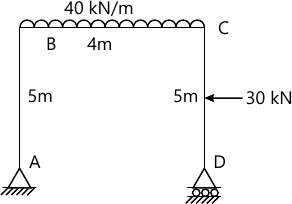7 M

3 (a) Find the slope at A and deflection under B for the beam shown in the fig.4 using Macauly's method. Take EI=3000 kNm2.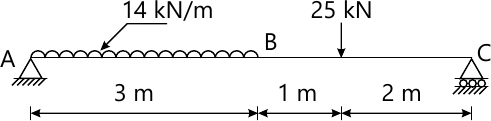7 M
3 (b) Draw I.L.D. for a member AB,AF & BF for the truss shown in the fig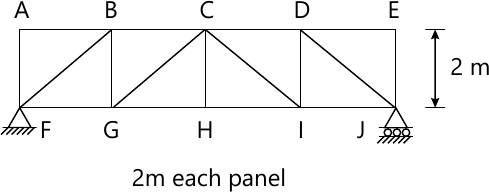7 M
3 (c) Find the slope and deflection at point A and B for the beam shown in the fig. using conjugate beam method. Take EI=3000 kN.m2.7 M
3 (d) A steel rod is 3 m long and 40 mm in diameter. An axial pull of 160 kN is suddenly applied to the rod. Calculate the instantaneous stress induced and also the instantaneous elongation produced in the rod. E=200 GN/m2
7 M

4 (a) A bar of diameter 20 mm and length of 2.2 m is attached with a collar at bottom. If the maximum stress developed is to be limited up to 180 N/mm2 , calculate the maximum value of weight that can be allowed to fall on the collar from 0.2m height. Assume E= 2×105 N/mm2 .
7 M
4 (b) A circular column has both end hinged with length of 6.0 m and diameter of 160 mm. If the yield strength of the material is 410 N/mm2 and Rankine's constant is 1/4800, calculate Euler's critical load and Rankine's critical load.
7 M
4 (c) A cylindrical shell 3 m long which is closed at the ends has an internal diameter of 1 m and wall thickness of 15 mm. Calculate the circumferential and longitudinal stresses induced if it is subjected to an internal pressure of 1.5 N/mm2 . Take E=2×105 Mpa & μ=0.3.
7 M
4 (d) A three hinged parabolic arch has a span 20m & central rise 3m. It carries a point load of 15 kN at 8 m from the left hinge. Calculate normal thrust, shear & B.M at a section 5 m from left end hinge. Also calculate maximum positive B.M & it's position. Draw B.M diagram.
7 M

5 (a) A rectangular column of width 200 mm and of thickness 150 mm carries a point load of 360 kN at an eccentricity of 10 mm on right side of minor axis and 15 mm above major axis . Determine the maximum and minimum stresses on the section.
7 M
5 (b) A cylindrical chimney 24 m high of uniform circular section is 4 m external dia. & 2m internal dia. It is subjected to a horizontal wind pressure of 1000 N/mm2. If the coefficient of wind pressure is 0.66 & unit wt. of masonry is 22 kN/m3 . Find the maximum & minimum stresses at the base of the section.
7 M
5 (c) Derive generalized formula for torsion of circular shaft (with usual notation)
$\dfrac{T}{Ip}=\dfrac{t}{r}=\dfrac{\theta}{L}$
7 M
5 (d) Determine the diameter of a solid shaft which will transmit 300 kW at 250 r.p.m. The maximum shear stress should not exceed 30 N/mm2 and twist should not be more than 1° in a shaft length of 2 m. Take modulus of rigidity = 10 5 N/mm2 .
7 M

More question papers from Structural Analysis - 1